Share

# Surface Tension

#### description

• Surface Energy and Surface Tension
• Surface Energy
• Angle of Contact
• Drops and Bubbles
• Capillary Rise
• Detergents and Surface Tension

Application of Surface Tension Ideas to Drops

#### notes

Surface Energy

• Surface energy is the excess energy exhibited by the liquid molecules on the surface compared to those inside the liquid.

• This means liquid molecules at the surface have greater energy as compared to molecules inside it.

• Suppose there is a tumbler and when we pour water in the tumbler, it takes the shape of the tumbler.

• It acquires free surface.

Case 1: When molecules are inside the liquid:-

• Suppose there is a molecule inside the water, there will be several other molecules that will attract that molecule in all the directions.

• As a result this attraction will bind all the molecules together.

• This results in negative potential energy of the molecule as it binds the molecule.

• To separate this molecule huge amount of energy is required to overcome potential energy.

• Some external energy is required to move this molecule and it should be greater than the potential energy.

• Therefore in order to separate this molecule a huge amount of energy is required.

Case2: When the molecules are at the surface:-

• When the molecule is at the surface, half of it will be inside and half of it is exposed to the atmosphere.
• For the lower half of the molecule it will be attracted by the other molecules inside the liquid.

• But the upper half is free. The negative potential energy is only because of lower half.

• But the magnitude is half as compared to the potential energy of the molecule which is fully inside the liquid.

• So the molecule has some excess energy, because of this additional energy which the molecules have at the surface different phenomenon happen like surface energy, surface tension.

• Liquids always tend to have least surface are when left to itself.

• As more surface area will require more energy as a result liquids tend to have least surface area.

Surface energy for two fluids in contact:

• Whenever there are two fluids, in contact, surface energy depends on materials of the surfaces in contact.

• Surface energy decreases if the molecules of the two fluids attract.

• Surface energy increases if molecules of the two fluids repel.

Surface Tension

• Surface tension is the property of the liquid surface which arises due to the fact that surface molecules have extra energy.

• Surface energy is the extra energy that the molecules at the surface have.

• Surface tension is the property of the liquid surface because the molecules have extra energy.

• Surface energy is defined as surface energy per unit area of the liquid surface.

• Denoted by ’S’.

Mathematically:-

• Consider a case in which liquid is enclosed in a movable bar.

• Slide the bar slightly and it moves some distance (‘d’).

• There will be increase in the area, (dl) where l=length of the bar.

• Liquids have two surfaces one on the bar and others above the bar. Therefore area=2(dl)

• Work done for this change =F x displacement.

• Surface tension(S)="Surface Energy"/"area"

• Or Surface Energy=S x area

• =S x 2dl

• Therefore S x 2dl =F x d

• "S" = "F"/"2d"

• Surface tension is the surface energy per unit area of the liquid surface.

• It can be also defined as Force per unit length on the liquid surface.

• Important: -At any interface (it is a line that separates two different medium) the surface tension always acts in the equal and opposite direction and it is always perpendicular to the line at the interface.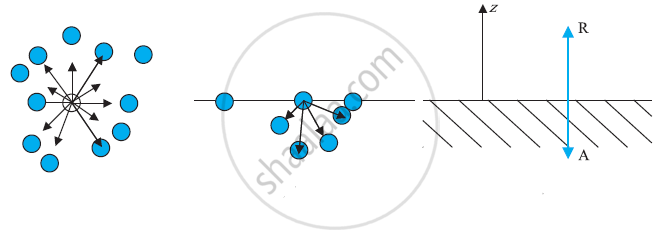a) A molecule inside a liquid. Forces on a molecule due to others are shown. The direction of arrows indicates the attraction of repulsion. (b) Same, for a molecule at a surface. (c) Balance of attractive (A) and repulsive (R) forces.

Surface tension and Surface energy: practical applications

1. Consider a molecule that is present completely inside the liquid and if it is strongly attracted by the neighboring molecules then the surface energy is less.

2. Consider a molecule that is present partially inside the liquid the force of attraction by the neighboring molecules is lesser as a result surface energy is more.

3. Consider a molecule whose very little part is inside the water so very small force of attraction by the neighboring molecules as a result of more surface energy.

• Conclusion: - A fluid will stick to a solid surface if the surface energy between fluid and solid is smaller than the sum of energies between solid-air and fluid-air.

This means Ssf( solid-fluid) < Sfa(fluid air) + Ssa(Solid air)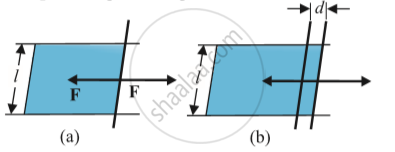Stretching a film:
(a) A film in equilibrium
(b) The film stretched an extra distance

Why does water stick to glass but Mercury doesn’t?

In case of water and glass, water sticks to glass because the surface energy of water and glass is less than the surface energy between water and air and between glass and air. "S.E"_(("w"-"g"))< "S.E"_(("w"-"a"))+ "S.E"_(("g"-"a"))

In case of mercury, surface energy between mercury and glass "S.E"_(("m"-"g")), surface energy between mercury and air "S.E"_((m-a)) and surface energy between air and glass "S.E"_(("a"-"g")).   "E"_(("m"-"g"))>"S.E"_(("m"-"a"))+"S.E"_(("a"-"g"))

How detergents work?

• Washing alone with the water can remove some of the dirt but it does not remove the grease stains. This is because water does not wet greasy dirt.

• We need detergent which mixes water with dirt to remove it from the clothes.

• Detergent molecules look like hairpin shape. When we add detergents to the water one end stick to water and the other end sticks to the dirt.

• As a result dirt is getting attracted to the detergent molecules and they get detached from the clothes and they are suspended in the water.

• Detergent molecules get attracted to water and when water is removed the dirt also gets removed from the clothes.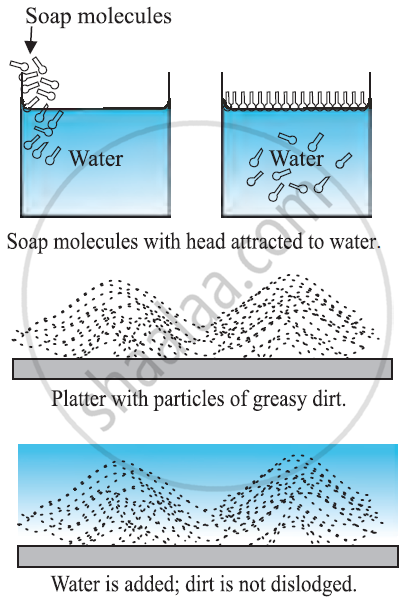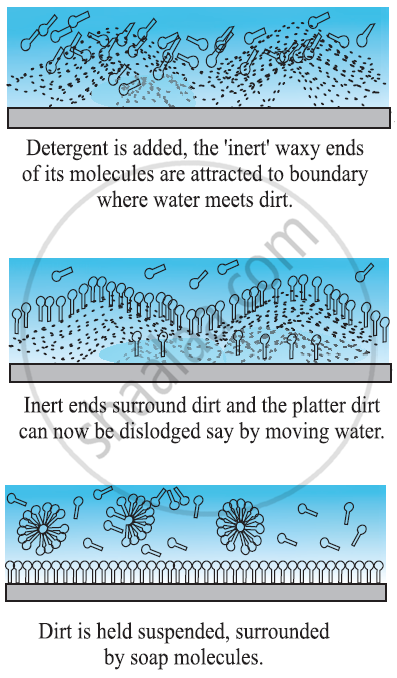Angle of Contact:-

• The angle of contact is the angle at which a liquid interface meets a solid surface.
• It is denoted by θ.

• It is different at interfaces of different pairs of liquids and solids.

• For example - Droplet of water on a lotus leaf. The droplet of water(Liquid) is in contact with the solid surface which is a leaf.

• This liquid surface makes some angle with the solid surface. This angle is known as angle of contact.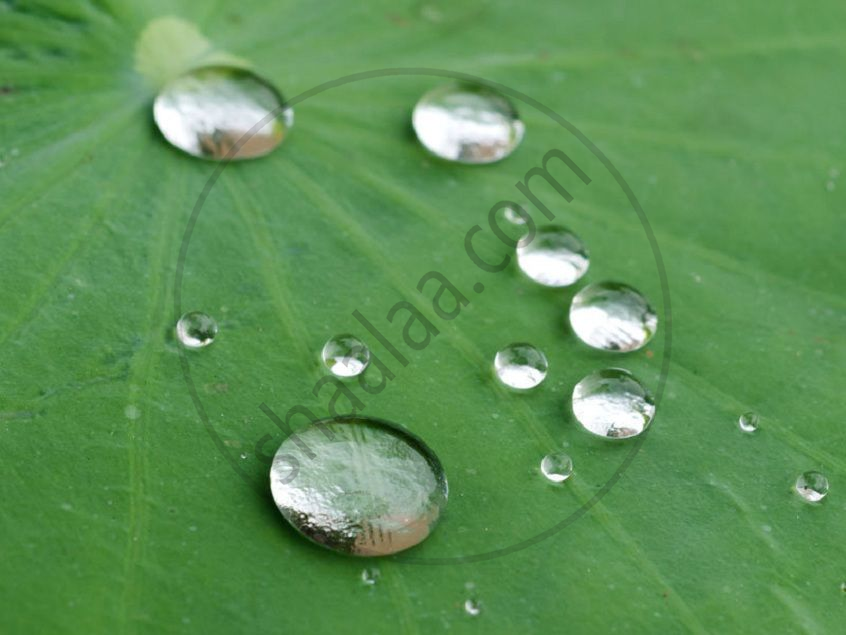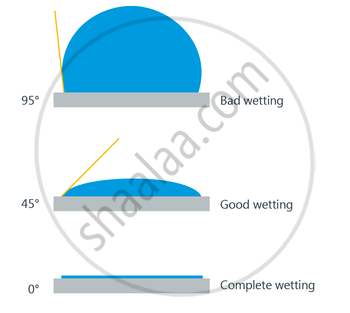Significance of Angle of Contact

• Angle of contact determines whether a liquid will spread on the surface of a solid or it will form droplets on it.

• If the Angle of contact is obtuse: then droplet will be formed.

• If the Angle of contact is acute: then the water will spread.

• Case1: When droplet is formed

• Consider we have a solid surface, droplet of water which is liquid and air.

• The solid liquid interface denoted by Ssl, solid air interface denoted by Ssa and liquid air interface denoted by Sla.

• The angle which Ssl makes with Sla. It is greater than the 900.

• Therefore droplet is formed.

Case 2: When water just spreads

• The angle which liquid forms with solid surface is less than 900.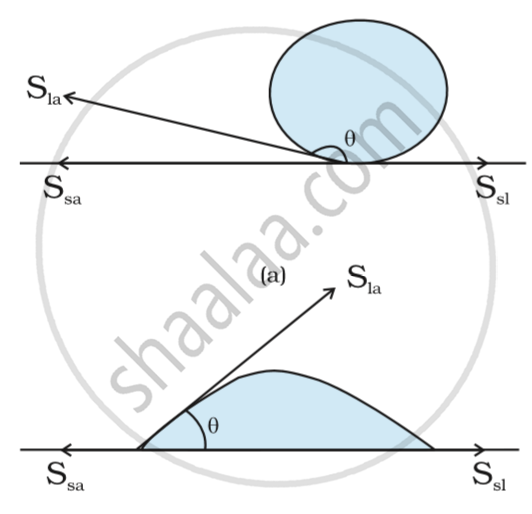Drops and Bubbles

Why water and bubbles are drops?

• Whenever liquid is left to itself it tends to acquire the least possible surface area so that it has the least surface energy so it has the most stability.

• Therefore for more stability, they acquire the shape of the sphere, as a sphere has the least possible area.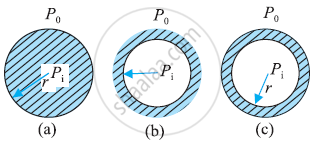Distinction between Drop, Cavity, and Bubble:

Drop: - Drop is a spherical structure filled with water:

There is only one interface in the drop.

The interface separates water and air.

Example: Water droplet.

Water droplets:

Cavity:- Cavity is a spherical shape filled with air.

In the surroundings there is water and in the middle, there is a cavity filled with air.

There is only one interface which separates air and water.

Example:- bubble inside the aquarium.

Bubble:- In a bubble there are two interfaces. One is air-water and another is water and air.

Inside a bubble there is air and there is air outside.

But it consists of a thin film of water.

Pressure inside a drop and a cavity

• The pressure inside a drop is greater than the pressure outside.

• Suppose there is a spherical drop of water of radius ‘r’ which is in equilibrium.

• Consider there is an increase in radius which is Δr.

Therefore extra surface energy = surface tension(S) x area

=S_"l"a xx 4pi(r+Deltar)^2-S_"l"a xx 4pir^2

after calculating

extra surface energy = 8piDeltarS_la

At equilibrium, extra surface energy = energy gain due to the pressure difference

8pir Deltar S_la = (P_i-P_o)4pir^2xxDeltar

where P_i = pressure inside the drop and P_o = pressure outside the drop.

After calculationP_i - P_o=(2S_(la))/r

Pressure inside a Bubble

• Pressure inside a bubble is greater than the pressure outside.

• As bubble has 2 interfaces, "P"_i-"P"_o=(4"S"_"la")/("r")
• This is probably why you have to blow hard, but not too hard, to form a soap bubble. A little extra air pressure is needed inside!

Capillary Rise

• In Latin the word capilla means hair.

• Due to the pressure difference across a curved liquid-air interface the water rises up in a narrow tube in spite of gravity.

• Consider a vertical capillary tube of circular cross section (radius a) inserted into an open vessel of water.

• The contact angle between water and glass is acute. Thus the surface of water in the capillary is concave. As a result there is a pressure difference between the two sides of the top surface. This is given by

("P"_i-"P"_o)=("2S"/r)="2S"/(a sec theta)=("2S"/a) cos theta      ...(i)

Thus the pressure of the water inside the tube, just at the meniscus is less than the atmospheric pressure.

consider the two points A and B. They must be at the same pressure.

"P"_0+"h"rho"g"="P"_i="P"_"A"     ...(ii)

Where rho is the density of water, and h is called the capillary.

By using eq (i) & (ii)

"h"rho"g" = ("P"_i-"P"_o)=(2"S" cos theta)/a

Therefore the capillary rise is due to surface tension. It is larger, for a smaller radius.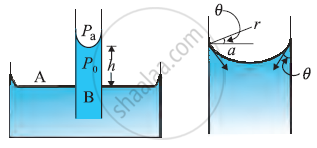Problem:- The lower end of a capillary tube of diameter 2.00 mm is dipped 8.00 cm below the surface of the water in a beaker. What is the pressure required in the tube in order to blow a hemispherical bubble at its end in the water? The surface tension of water at temperature of the experiments is 7.30 ×10-2 Nm-1. 1 atmospheric pressure = 1.01 × 105 Pa, density of water = 1000 kg/m3, g = 9.80 m s-2.Also, calculate the excess pressure.

The excess pressure in a bubble of gas in a liquid is given by "2S"/r, where 'S' is the surface tension of the liquid-gas interface. As there is only one liquid surface, therefore using the formula pressure is "4S"/r. The radius of the bubble is 'r'. Now the pressure outside the bubble Po equals atmospheric pressure plus the pressure due to 8.00 cm of water column. That is

"P"_o=(1.01xx105"Pa"+0.08"m"xx1000"kgm"^-3xx9.80"ms"^-2)

= 1.01784 xx 10^5  "Pa"

Therefore, the pressure inside the bubble is

"P"_i="P"_o + "2S"/"r"

1.01784 xx 10^5Pa +((2xx7.3xx10^-2)/10^-3 )

=(1.01784+0.00146)xx10^5"Pa"

=1.02xx10^5"Pa" where the radius of the bubble is taken to be equal to the radius of the capillary tube since the bubble is hemispherical.

### Shaalaa.com

Angle Of Contact [00:28:02]
S
1
0%

S